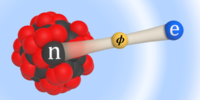Physical Review Letters ( IF 8.385 ) Pub Date : 2020-09-15 , DOI: 10.1103/physrevlett.125.123003
Cyrille Solaro; Steffen Meyer; Karin Fisher; Julian C. Berengut; Elina Fuchs; Michael DrewsenWe perform high-resolution spectroscopy of the $3d\text{\hspace{0.17em}}{{}^{2}\mathrm{D}}_{3/2}-3d\text{\hspace{0.17em}}{{}^{2}\mathrm{D}}_{5/2}$ interval in all stable even isotopes of ${{}^{A}\mathrm{Ca}}^{+}$ ($A=40$, 42, 44, 46, and 48) with an accuracy of $\sim 20\text{\hspace{0.17em}}\text{\hspace{0.17em}}\mathrm{Hz}$ using direct frequency-comb Raman spectroscopy. Combining these data with isotope shift measurements of the $4s\text{\hspace{0.17em}}{{}^{2}\mathrm{S}}_{1/2}↔3d\text{\hspace{0.17em}}{{}^{2}\mathrm{D}}_{5/2}$ transition, we carry out a King plot analysis with unprecedented sensitivity to coupling between electrons and neutrons by bosons beyond the standard model. Furthermore, we estimate the sensitivity to such bosons from equivalent spectroscopy in ${\mathrm{Ba}}^{+}$ and ${\mathrm{Yb}}^{+}$. Finally, the data yield isotope shifts of the $4s\text{\hspace{0.17em}}{{}^{2}\mathrm{S}}_{1/2}↔3d\text{\hspace{0.17em}}{{}^{2}\mathrm{D}}_{3/2}$ transition at 10 parts per billion through combination with recent data of Knollmann, Patel, and Doret [Phys. Rev. A 100, 022514 (2019)].

down
wechat
bug